Key Stage 2 Outcomes 2017 – 2019

Key Stage 2 Outcomes for the 2019-20 were withdrawn from statutory publication due to the Covid-related closure of schools. The data below represents the previous three years of published pupil outcomes.

Statutory assessments for the academic year 2020-21 were also withdrawn following the national COVID lockdown.

Reading: Percentage of Year 6 pupils at or above standard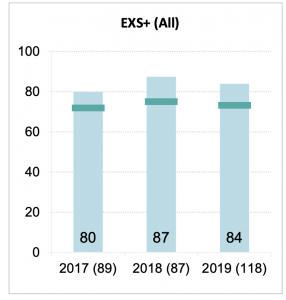Expected Standard              2017             2018          2019

Denbigh Primary School.        80%.             87%.          84%

LA Schools (Ave).                        65%.             70%.          68%

National (Ave).                             72%.             75%.          73%

Greater Depth (exceeding)    2017             2018          2019

Denbigh Primary School.        23%.            46%.          42%

LA Schools (Ave).                         18%.             21%.          22%

National (Ave).                              25%.             28%.          27%

Mathematics: Percentage of Year 6 pupils at or above standard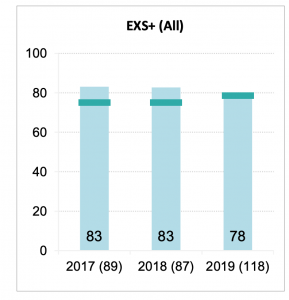2017             2018          2019

Denbigh Primary School.        83%.             83%.          79%

LA Schools (Ave).                        73%.             73%.          75%

National (Ave).                             75%.             75%.          79%

Greater Depth (exceeding)    2017             2018          2019

Denbigh Primary School.        19%.            31%.          42%

LA Schools (Ave).                        20%.             21%.          26%

National (Ave).                              23%.             24%.          27%

Writing: Percentage of Year 6 pupils at standard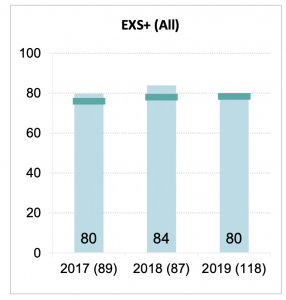2017             2018          2019

Denbigh Primary School.        80%.             84%.          80%

LA Schools (Ave).                        72%.             75%.          73%

National (Ave).                              76%.             78%.          78%

Greater Depth (exceeding)    2017             2018          2019

Denbigh Primary School.          14%.            20%.          21%

LA Schools (Ave).                         11%.             16%.          17%

National (Ave).                               18%.             20%.          20%

GPS (Grammar, Punctuation and Spelling): Percentage of Year 6 pupils at  or above standard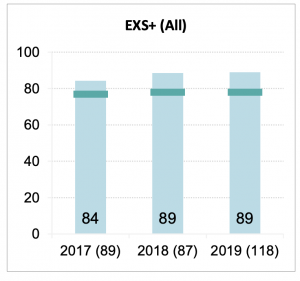2017             2018          2019

Denbigh Primary School.         84%.             89%.          89%

LA Schools (Ave).                          79%.             77%.          77%

National (Ave).                               77%.             78%.          78%

Greater Depth (exceeding)       2017             2018          2019

Denbigh Primary School.          64%.            64%.          71%

LA Schools (Ave).                          35%.             37%.          38%

National (Ave).                                31%.             34%.          36%

RWM Combined (Pupils achieving at standard in Reading, Writing and Mathematics)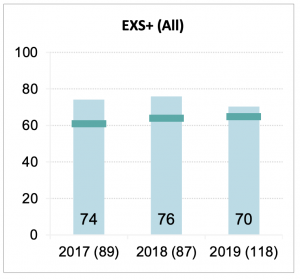2017             2018          2019

Denbigh Primary School.         74%.             76%.          70%

LA Schools (Ave).                         55%.             59%.          60%

National (Ave).                              61%.             64%.          65%

Greater Depth (exceeding)    2017             2018          2019

Denbigh Primary School.        8%.              13%.           16%

LA Schools (Ave).                         5%.               8%.             9%

National (Ave).                              9%.              10%.           11%

The link to the DfE’s Performance Data overview for Denbigh Primary School can be found here.